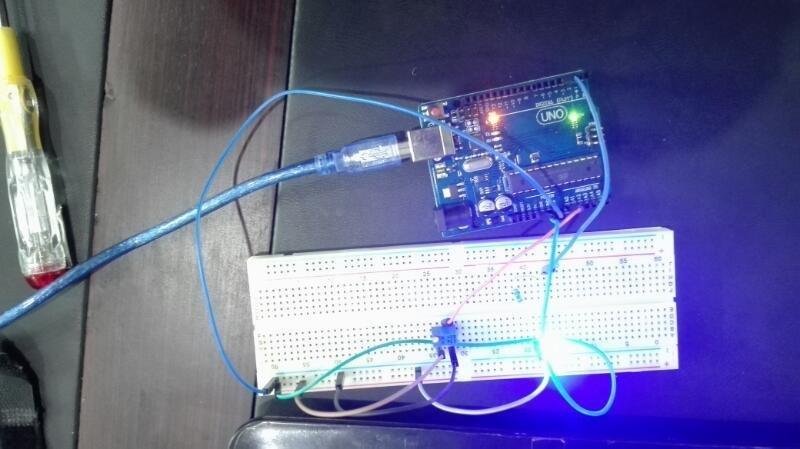# Analog voltage reading using Arduino UNO R3

Analog voltage reading using Arduino UNO R3, In this article I have discussed how to use analog to digital converter channels of Arduino UNO R3? How to read analog voltage using this board? How to these channels to communicate with analog world? Arduino is a small microcomputer system which don’t understand analog values. Every microcomputer system deals with digital binary numbers in the form of 0’s and 1’s. So Arduino can not understand analog values without the use of analog to digital converters. Many microcontrolllers have built in analog to digital converters. Arduino UNO R3 also have five built in analog to digital converter channels. Its mean it can read up to five analog voltage values or can be interfaced with five analog output sensors.#### Application of Analog to digital converters in embedded system :

In embedded system world there are enormous applications of ADC. In your home appliances, there are many applications of embedded system which use analog to digital converters. For example, In oven temperature sensor is used to measure temperature. Temperature sensor give output in the form of analog voltage. So a analog to digital converter is used in oven embedded system to interface temperature sensor with microcontroller. Some applications of ADC are given below :

• Analog sensors interfacing with Arduino like temperature, humidity, moisture, light, pressure and velocity sensors.
• Analog voltage and current measurements
• and many other applications

#### How to use ADC channels of Arduino UNO R3 :

Unlike other microcontrollers, to use Analog to digital converter channels of Arduino you only need yo use one function to read analog voltage. But in other microcontrollers, you have to write complete code by using ADC control registers. But Arduino provide rich libraries, ADC library can be easily used to read analog readings. Figure below shows, Arduino UNO R3 have five ADC channels. Function analogRead(channel number) is used to read analog voltage where channel number is used to specified which channel you are using to read analog voltage.

#### Circuit diagram :

To understand analog to digital converter of Arduino R3, Led is connect with pin number 3 and a variable resistor is connected with channel A0 of Arduino UNO R3 and variable resistor one side is connect with 5 volt and other side is connected with ground. Third terminal of variable resistor is connected with channel A0 of Arduino. Circuit shown below is work in such a way that when voltage read by channel A0 become greater than 3 volt LED connected to pin 3 glows and when voltage is less than 3 volt light remain turn off.

As shown in above circuit diagram, when voltage is greater than 3 volt led glows and voltmeter is connected with variable resistor just for checking of voltage.

#### Code :

```Code written below is self explanatory. But if you still have any issue, your comments are welcome.

int sensorPin = A0; // select the input pin for the potentiometer
int ledPin = 3; // select the pin for the LED
int sensorValue = 0; // variable to store the value coming from the sensor

void setup() {
// declare the ledPin as an OUTPUT:
pinMode(ledPin, OUTPUT);
}

void loop()

{
// read the value from the sensor:
sensorValue = sensorValue * 5/1023 ; //its convert digital value back into voltage
// turn the ledPin on
if(sensorValue >= 3 )
digitalWrite(ledPin, HIGH);
else
digitalWrite(ledPin, LOW);
}```

### 5 thoughts on “Analog voltage reading using Arduino UNO R3”

1. why you have multiply “Sensor Value *5/1023” ?

2. CAn I use this same method but with 2 output so If it reads below 3 it sends out a signal through output1 and if it reads more than 3v it sends out a signal through output2 ?

• Hi Marc,
You can use two outputs using same method but you need one LED for another pin.
the program is then modified as.,
at beginning of the program, you need to define one more pin for second LED like
int ledpin2 = 4; // it uses pin 4 for second LED.
in setup, you need to add
pinMode (ledpin2, OUTPUT);
in loop, you need to replace ledpin with ledpin2 in else case.
that’s it you are done.

3. I required coding for dispaly the anlog voltsge on display